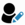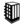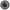Find Fibonacci first n numbers using recursion ?# Search Interview Questions3348 questions in repository.There are more than 200 unanswered questions.Have a video suggestion.
Click Correct / Improve and please let us know.
Label / Company      Label / Company / TextSubmit Question

Java - Interview Questions and AnswersQ1. Find Fibonacci first n numbers using recursion ?Core Java2018-05-13 19:42:03Ans. public int fibonacci(int n) {
if(n == 0)
return 0;
else
if(n == 1)
return 1;
else
return fibonacci(n - 1) fibonacci(n - 2);
}Help us improve. Please let us know the company, where you were asked this question :LikeDiscussCorrect / ImproveAsked in 1 CompaniesRelated QuestionsWhat will happen if we don't have termination statement in recursion ?Write an Algorithm for Graph Traversal ? The Graph has a loop.Write a Program to print factorial of a number using recursionWrite a program to print sum of numbers between the start and end number

For example - Passing start number as 2 and end number as 10, it should print 2+3+4+5+6+7+8+9+10 = 54Write a program using Recursion to print multiplication of numbers between the start and end number

For example - Passing start number as 2 and end number as 10, it should print 2*3*4*5*6*7*8*9*10 = 3628800What is recursion in Java ?What will happen if we don't have termination statement in recursion ?Which of the two - iteration or recursion - is slower ?Write a Program to print fibonacci series using recursion.

Subscribe to Java News and Posts. Get latest updates and posts on Java from Buggybread.com